# SCIENTIFIC NOTATION WHAT DOES SCIENTIFIC NOTATION MEAN By

• Slides: 8SCIENTIFIC NOTATION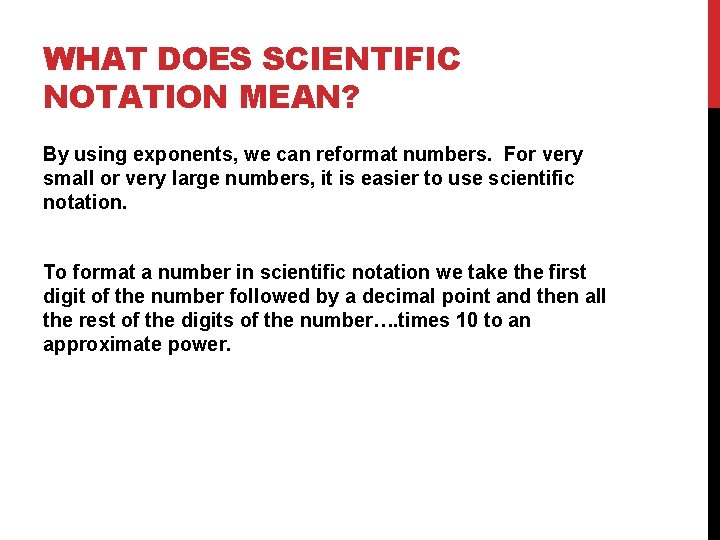WHAT DOES SCIENTIFIC NOTATION MEAN? By using exponents, we can reformat numbers. For very small or very large numbers, it is easier to use scientific notation. To format a number in scientific notation we take the first digit of the number followed by a decimal point and then all the rest of the digits of the number…. times 10 to an approximate power.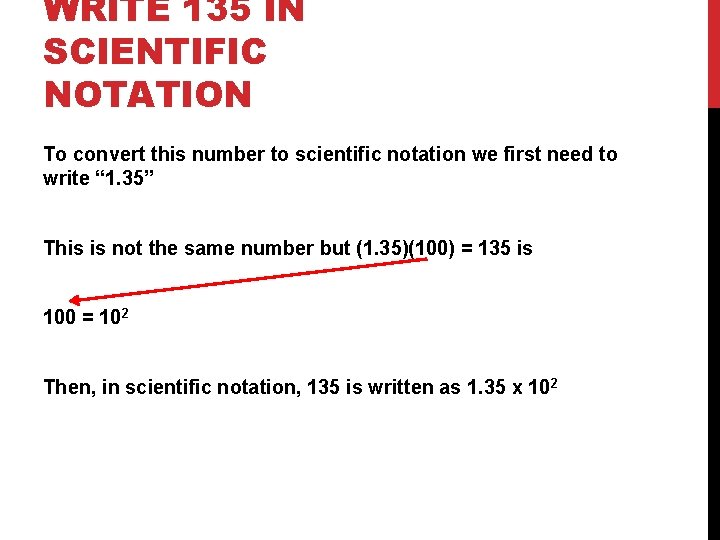WRITE 135 IN SCIENTIFIC NOTATION To convert this number to scientific notation we first need to write “ 1. 35” This is not the same number but (1. 35)(100) = 135 is 100 = 102 Then, in scientific notation, 135 is written as 1. 35 x 102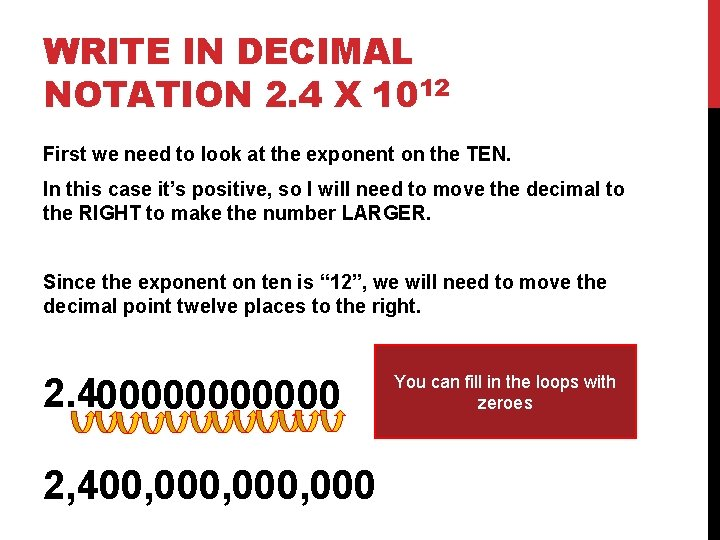WRITE IN DECIMAL NOTATION 2. 4 X 1012 First we need to look at the exponent on the TEN. In this case it’s positive, so I will need to move the decimal to the RIGHT to make the number LARGER. Since the exponent on ten is “ 12”, we will need to move the decimal point twelve places to the right. 2. 4000000 2, 400, 000, 000 You can fill in the loops with zeroesWRITE 0. 000543 IN SCIENTIFIC NOTATION First, you need to move the decimal point of a number until the new form is a number from 1 UP TO 10. In this case I need to move the decimal point between the ‘ 5” and “ 4”. 0. 000543 You’ve moved the decimal FOUR times to the right. When you move the decimal point to the right your exponent is negative. 5. 43 x 10 -4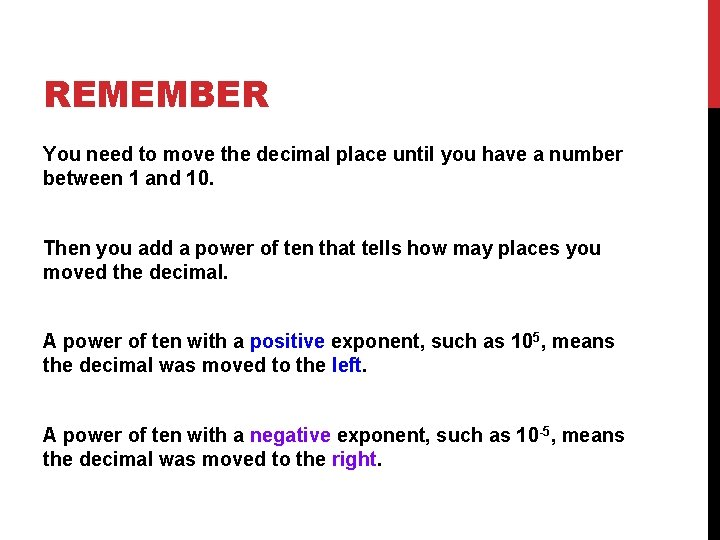REMEMBER You need to move the decimal place until you have a number between 1 and 10. Then you add a power of ten that tells how may places you moved the decimal. A power of ten with a positive exponent, such as 105, means the decimal was moved to the left. A power of ten with a negative exponent, such as 10 -5, means the decimal was moved to the right.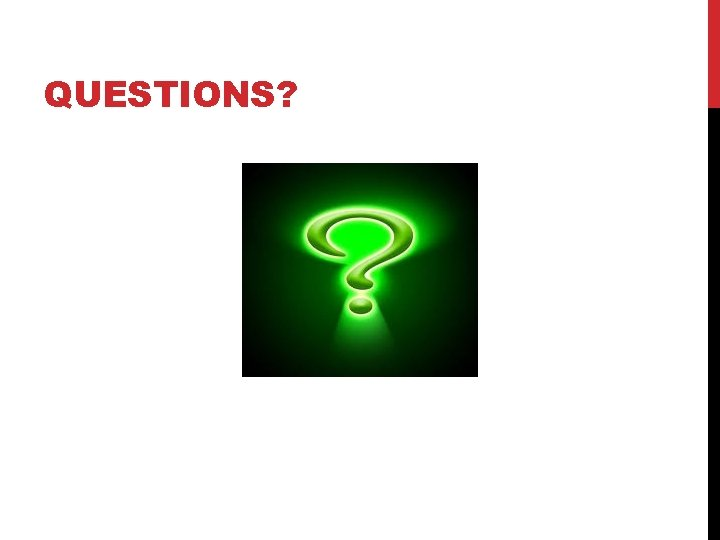QUESTIONS?REFERENCES www. edgenuity. com http: //www. factmonster. com/ipka/A 0876783. html http: //www. purplemath. com/modules/exponent 3. htm